Courses

# Sample Question Paper - 7 Class 9 Notes | EduRev

## Class 9 : Sample Question Paper - 7 Class 9 Notes | EduRev

The document Sample Question Paper - 7 Class 9 Notes | EduRev is a part of the Class 9 Course Sample Papers For Class 9.
All you need of Class 9 at this link: Class 9

SECTION - A

Q.1. Velocity-time graphs for two particles P and Q have been shown here. Which of the following statement about them is correct?
(а) Velocities of P and Q are equal.
(b) Accelerations of P and Q are equal.
(c) Acceleration of P is more than that of Q.
(d) Acceleration of Q is more than that of P.
Ans.
(d) Slope of velocity-time graph gives the value of acceleration. As slope of v-t graph for Q is more than that for P, hence acceleration of Q is more than that of P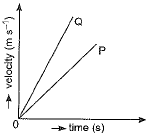Q.2. "Tyndall Effect can be observed when sunlight passes through the canopy of dense forest." Explain how this occurs.
Ans.
This is because forest contains mist which in turn contains tiny droplets of water that act as particles of colloid dispersed in air.

Q.3. Which among the following is exclusively marine?
(a) Porifera
(b) Echinodermata
(c) Mollusca
(d) Pisces
OR
Pteridophyta do not have
(a) Root
(b) Stem
(c) Flowers
(d) Leaves
Ans.
Echinodermata
Echinodermata are spiny skinned marine animals. Porifera consist of sponges. Most sponges are marine but few fresh water forms are also seen like Spongilla. Molluscs are marine as well as terrestrial forms. Pisces are found in both marine water, (e.g., dog fish) and freshwater (e.g., Rohu).
OR
(c) Flowers
Pteridophytes do not produce seeds, or are seedless plants and have no flowers.

Q.4. The sensation of sound persists in human brain for about
(a) 1s
(b) 1/10 s
(c) 1/16 s
(d) 1 minute
Ans.
(b) The sensation of sound persists in human brain for about 1/10 second.

Q.5. A particle accelerates from rest at a constant rate for sometime and attains a constant velocity of 8 ms-1. Afterwards it decelerates with a constant rate an d comes to rest. If the total time taken is 4 second, the distance travelled is:
(a) 32 metre (b) 16 metre (c) 4 metre (d) insufficient data
OR
The velocity-time graph of a body has a negative slope. The body is undergoing:
(a) uniform acceleration
(b) uniform retardation
(c) variable acceleration
(d) variable retardation
Ans.
(b) Or (b)

Q.6. Two substances, A and B were made to react to form a third substance, A2B according to the following reaction 2A + B → A2B. Which of the following statements concerning this reaction are incorrect?
(i) The product A2B shows the properties of substances A and B.
(ii) The product will always have a fixed composition.
(iii) The product so formed cannot be classified as a compound.
(iv) The product so formed is an element.
(a) (i), (ii) and (iii)
(b) (ii), (iii) and (iv)
(c) (i), (iii) and (iv)
(d) (ii), (iii) and (iv)
Ans.
(c) (i), (iii) and (iv)
A2B is a compound made up of two elements A and B in a fixed ratio. The properties of a compound (e.g., A2B) are entirely different from those of its constituent elements (e.g., A and B). The composition of a compound is always fixed.

Q.7. Mass can neither be created nor destroyed. This is the statement of
(a) Law of constant proportion
(b) Dalton’s law
(c) Law of conservation of mass
Ans.
(c) Law of conservation of mass

Q.8. Which of the following are exotic breeds?
(i) Prawn (ii) Jersey (iii) Brown Swiss (iv) Jersey Swiss
(a) (i) and (iii) (b) (ii) and (iii) (c) (i) and (iv) (d) (ii) and (iv)
OR
Poultry farming is undertaken to raise following:
(i) Egg production (ii) Feather production (iii) Chicken meat (iv) Milk production
(a) (i) and (iii) (b) (i) and (ii) (c) (ii) and (iii) (d) (iii) and (iv)
Ans.
(b) Or (a)

Q.9. The commercial unit of energy consumption in households, industries and commercial establishments is
(a) Joule (b) Watt (c) kW (d) kWh (kilowatt hour)
Ans.
(d) kWh

Q.10. Identify a non-membranous organelle from the following:
(a) Lysosome (b) Ribosome (c) Chloroplast (d) Golgi apparatus
Ans.
(b) Chloroplast and golgi bodies are double membranous organelle whereas lysosomes are single membrane spherical organelle having hydrolytic enzymes.

Q.11. Which of the following tissues has dead cells?
(a) Parenchyma
(b) Sclerenchyma
(c) Collenchyma
(d) Epithelial tissue
Ans.
(b)

Q.12. How many atoms are there in 1 gm of hydrogen?
OR
Interpret the number of moles of oxygen atoms in PO3-4.
Ans.
6.022 x 1023 atoms or one mole.
OR
Each mole of phosphate ion possesses 4 moles of oxygen atoms as represented by the formula.

Q.13. During a free fall, the acceleration of the falling object is directly proportional to its mass.
Ans.
False - During a free fall the acceleration of the falling object is independent of its mass and has a constant value g = 9.8 m s-2.

Q.14. For question numbers 13 and 14, two statements are given-one labelled Assertion (A) and the other labelled Reason (R). Select the correct answer to these questions from the codes (i), (ii), (iii) and (iv) as given below.
(i) Both A and R are true and R is correct explanation of the assertion.
(ii) Both A and R are true but R is not the correct explanation of the assertion.
(iii) A is true but R is false.
(iv) A is false but R is true.
Assertion: While sharpening a knife, sparks fly off tangentially from the grinding stone, due to inertia of direction.
Reason: The inherent property of a body by virtue of which it cannot change its direction of motion is called inertia of direction. (i) A (ii) B (iii) C (iv) D
Ans.
(i)

SECTION - B

Q.15. A car moves with a speed of 30 km h-1 for half an hour, 25 km h-1 for one hour and 40 km h-1 for two hours. Calculate the average speed of the car.
Ans. Distance travelled by the car in time t1 = 1/2 h with a speed v1 = 30 km h-1 is: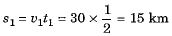Distance travelled by the car in time t2 = 1 h with a speed v2 = 25 km h-1 is:
s2 = v2t2 = 25 x 1 = 25 km
and the distance travelled by the car in time t3 = 2 h with a speed v3 = 40 km h-1 is:
s3 = v3t3 = 40 x 2 = 80 km
∴ Average speed of car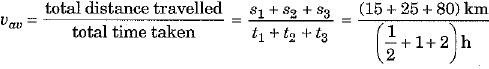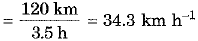Q.16. How will you separate a mixture of naphthalene balls powder and common salt? Draw a neat and labelled diagram to show that process.
OR
Both smoke and fog are aerosols. In what way they are different?
(b)
How do sol and get differ from each other? Give one example for each.
Ans.
Take the mixture in a china dish. Cover it with an inverted funnel. Close the stem of the funnel with cotton ball. Heat the mixture.
Naphthalene powder forms vapours and gets condensed on the inner walls of the funnel. Common salt is left in the china dish.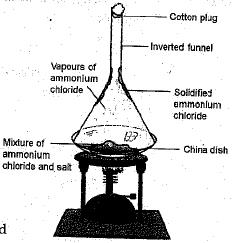OR
(a) Smoke and fog differ in dispersed phase. In smoke, dispersed phase is solid and in fog, dispersed phase is liquid while the dispersion medium is gas in both of them.
(b) Sol → Dispersed phase → solid Example → mud
Dispersion medium → liquid
Gel → Dispersed phase → liquid Example → butter/cheese
Dispersion medium → solid

Q.17. (i) Do all bodies immersed in a given fluid experience the same buoyant force? Explain.
(ii) A 100 cm3 block has a mass of 395 gm. Find its relative density. (Density of water = 1 gm/cm3)
OR
Give the mathematical formula that -relates thrust and pressure. Define 1 pascal.
Calculate the thrust and pressure exerted by a block of 500 N on the surface of table if the surface area in contact is 2.5 m2.
Ans.
(i) No, all the bodies immersed in the given fluid will displace different weights of the fluid, and hence, the buoyant force experienced by each of the bodies will be different.
(ii)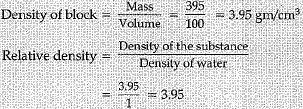OR
Pressure = Thrust/Area
1 pascal is the pressure acting on a surface area 1 Square metre by a thrust of X newton.
Thrust = Weight of body = 500 N
Pressure = thrust/area = 500/2.5 = 200 Pascal

Q.18. Air is a chemical compound or a mixture? Support your answer by suitable facts.
Ans.
Air is a mixture having nitrogen and oxygen as the main components.
The following facts support that air is a mixture:
(i) The air contains various gases the proportion of which varies from place to place. For example, the air around the city has less oxygen than the air around a village or an open land.
(ii) When the gases such as oxygen, nitrogen and carbon dioxide are mixed to obtain a sample of air, no heat, light or electricity is evolved or absorbed.
(iii) The various gases found in air can easily be separated by fractional distillation of liquid air.
(iv) Air retains the properties of its constituents.

Q.19. Differentiate between striated, unstriated and cardiac muscles on the basis of their structure and site/ location in the body.
Ans.

 Characteristics Striated muscles Unstriated muscles Cardiac muscles Structure (i) These muscles are made-up of long, cylindrical, unbranched and multinucleate cells.(ii) These muscles show alternate light and dark bands or striations when stained appropriately. These muscles are madeup of long uninucleate cells with pointed ends (spindle shaped cells).These muscles do not show straitions. These muscles are madeup of cells which are cylidrical, branched and uninucleate.These muscles show frint cross striations. Site/location These muscles occur in the limbs and are mostly attached to bones to help in body movement. These muscles are present mostly in the walls of tubular organs such as alimentary canal, blood vessels, ureters and bronchi of the lungs. These are also found in the iris of the eye. These muscles are found in the walls of heart only.

Q.20. Identify the type of tissues in the following:
(i) Vascular bundle
(ii) Inner lining of the intestine
(iii) Lining of kidney tubule
(iv) Iris of the eye
(v) Muscles of the heart
(vi) Bronchi of lungs.
Ans.
(i) Complex tissues
(ii) Columnar epithelium
(iii) Cuboidal epithelium
(iv) Involuntary muscular tissues
(v) Cardiac musdes
(vi) Ciliated cuboidal epithelium.

Q.21. How will you separate dyes in black ink using chromatography?
Ans.
The following steps are involved in the process:
(i) Take a thin strip of filter paper.
(ii) Draw a line on it with the help of a pencil nearly 3 cm above the lower edge.
(iii) Put a small drop of the given ink with the help of a pen at the centre of the line and let it dry.
(iv) Lower the filter paper into a glass/jar/ beaker containing water in such a way that the ink drop remains just above the water level. Leave it undisturbed.
(v) Water rises up on the filter paper. Watch it carefully and note that the ink drop splits up into its constituents.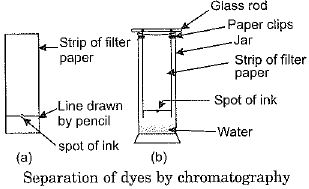It indicates that the dye in black ink is a mixture of different components.

Q.22. Define 'acceleration due to gravity, of earth'. Does the acceleration produced in a freely falling body depend on the mass of the body? Justify your answer mathmetically.
OR
A boy drops a ball from d cliff 122.5 m high. Find:

(i) How long does it take the ball to fall to the ground?
(ii) How far does it fall in the first three seconds?
(iii) How fast is it going at the end of the three second?
Ans.
It is the acceleration with which bodies fall towards the surface of the earth.
Acceleration produced in a freely falling body does not depend upon the mass of the body.
According to second law of motion
f = mg ...(i)
By Newton's law of gravitation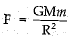...(ii)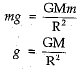Where M is the mass of the earth and R is the radius of the earth.
This shows that acceleration produced in a freely falling body is independent of mass.
OR
Distance travelled by the ball, S = 122.5 m
Initial velocity, u = 0
Acceleration, a = g = 9.8 ms-2
(i) If t is the time taken by the ball to fall on the ground, then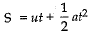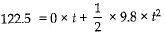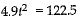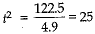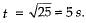(ii) Distance travelled by the ball in first three seconds is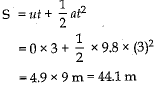(iii) Velocity of the ball at the end of three seconds is
v = u + at
= 0 + 9.8 x 3 = 29.4 m s-1

Q.23. The distance moved by a student at different intervals of time, while walking to school, is given in the table below: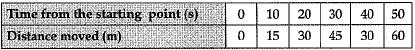Draw the distance-time graph for the motion of the student indicating the scale chosen. What does the shape of the graph suggest about the type of motion?
Ans.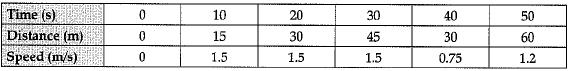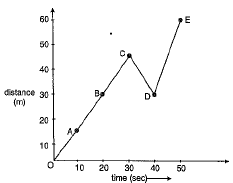The graph indicates non-uniform motion. From point A to point C, the student moves with constant speed and from point C to D, he is reducing the speed and from D to E again constant speed and positive.

Q.24. What is the difference between the plasma membrane and cell wall ? Give the functions of each one.
OR
Differentiate between hypertonic, hypotonic and isotonic solutions.
Ans.
Difference between plasma membrane and cell wall: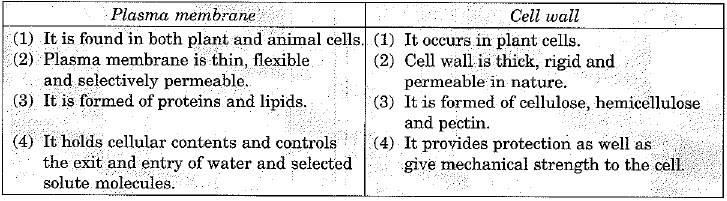OR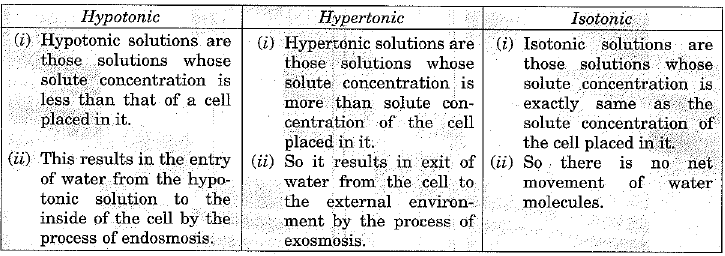SECTION - C

Q.25. (i) Give reason o f the following:
(a) A footballer kicks a ball, which rolls on the ground and after covering some distance comes to rest.
(b) Only the carrom coin at the bottom of a pile is removed when a fast moving striker hits it.
(ii) A force of 5 N produces an acceleration of 8 m s-2 in mass m1 and an accelera-tion of 24 m s-2 in mass m2. What acceleration would it give if both the masses are tied together?
OR
(а) State the reason why a bullet of small mass fired from a gun kills a person.
(b) A bullet of mass 4 g fired with a velocity of 50 m s-1 enters a wall up to a depth of 10 cm. Calculate the average resistance offered by the wall.
(c) How will the depth of penetration into the wall change if a bullet of mass 5 g strikes against it with a velocity of 40 m s-1? Give reason to justify your answer.
Ans. (i) (a) When football is rolling on the ground, a force of friction acts on it due to ground in a direction opposite to its motion. As a result, the motion of football gets slowed down and after covering some distance it comes to rest.
(b) The carrom coin at the bottom of a pile comes in a state of motion due to force exerted by the striker on it. However, other coins of pile remain intact due to their inertia of rest.
(ii) Here force f = 5 N, acceleration α= 8m s-2 and acceleration α= 24 m s-2.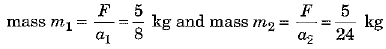When both the masses are tied together, the total mass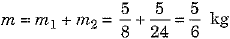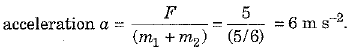OR
(a) Although mass of a bullet is small but its momentum is very large on account of its extremely large velocity. When a bullet hits a person this large momentum is transferred to the person within a very short time and thus produces a large force and may kill the person.
(b) Here mass of bullet m = 4 g = 0.004 kg, initial velocity u = 50 m s-1, final velocity v = 0 and distance covered in wall s = 10 cm = 0.1 m.
As per relation v2 - u2 = 2as, the acceleration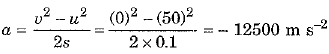∴ Average resistance force offered by wall F = ma = 0.004 x (- 12500) = - 50 N
(c) Depth of penetration will decrease because initial velocity of bullet is less but magnitude of retardation due to the wall remains unchanged.

Q.26. Write the chemical formulae of the compounds formed by the following ions:
(a) Mg2+ and S2-
(b) Cu2+ and OH
(c) Al3+ and Br-
Name the compounds formed in each case.
Ans.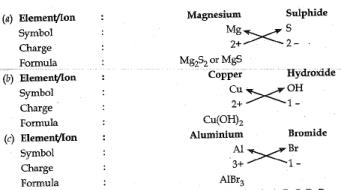Q.27. Explain giving reasons:
(i) Balanced diet is necessary for maintaining healthy body.
(ii) Health of an organism depends upon the surrounding environmental conditions.
(iii) Our surrounding areas should be free of stagnant water.
(iv) Social harmony and good economic conditions.are necessary for good health.
Ans.
(i) Food is necessary for the growth and development of the body. Balanced diet provides raw materials and energy in appropriate amount in the form of protein, carbohydrates, fats, minerals etc. which, in turn, are essential for the proper growth and functioning of the healthy body. Lack of single nutrient may cause deficiency diseases.
(ii) Surrounding environmental conditions play an important role in the maintenance of health. For example, we feel depressed if surroundings are dirty or polluted or if garbage is not collected or properly disposed or drains are not cleaned or if water collects in the streets or open spaces. This unclean surrounding causes the entry of germs via air, water, food or vectors and makes the person unhealthy.
(iii) Stagnant water provides a breeding ground for several disease-causing insects, especially mosquitoes. Mosquito acts as a vector for several disease like malaria, dengue, etc. So, if we keep our surroundings clean and free from stagnant water, then these vectors will not find any place for breeding and automatically their population will decrease. This in turn, will prevent the spread of insect-borne diseases.
(iv) Human beings live in societies and different localities like villages or cities, which determines the social and physical environment, both are to be kept in harmony. Public cleanliness is important for individual health. A lot of money is required for maintaining better living conditions. We need good food for healthy body  and for this we have to earn more. For the treatment of diseases also, one has to be in good economic condition.

Q.28. What is a meristematic tissue? State its different types. Show their location in a diagram of plant body. Give classification of meristems.
Ans.
Meristematic tissue are thin walled, compactly arranged undifferentiated cells that have the potential to divide and form new cells. They are classified into three subtypes based on their location.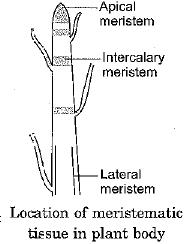(a) Apical meristem - It occurs in the growing tips of stem and roots. It increases the length of the stem and root.
(b) Lateral meristem - It occurs on the sides, both in stem and root. It is responsible for increase in the girth or diameter of root and stem.
(c) Intercalary meristem - It occurs at the base of leaves, internodes and is responsible for the growth of branches and leaves.

Q.29. A stone is dropped from the top of a tower 500 m high into a pond of water at the base of the tower. When is the splash heard at the top? Given, g = 10 m s-2 and speed of sound = 340 m s-1.
Ans.
For the dow nward jou rn ey of stone.
Initial velocity (u) = 0
Distance i.e., height of tower (S) = 500 m
Time of fall (t1) = ?
Acceleration due to gravity (g) = 10 m s-2
We know;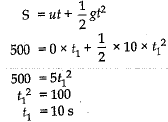For the sound travelling upward.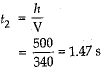Time required to hear splash = t+ t2 = 10 + 1.47 = 11.47 s

Q.30. (a) What are the consequences of the following conditions?
(i) A cell having higher water concentration than the surrounding medium.
(ii) A cell having lower water concentration than the surrounding medium.
(iii) A cell having equal water concentration to its surrounding medium.
(b) Name the materials of, which the cell membrane and cell wall are composed of.
OR
Draw a neat diagram of an animal cell and label any four parts of it.
Ans.
(a) (i) When a cell possess higher water concentration than the surrounding medium then exosmosis occurs in the cell due to difference in concentration and cell shrinks.
(ii) When a cell has low water concentration than surrounding medium then endosmosis occurs that results in the swelling of the cell.
(iii) A cell having equal water concentration to its surrounding medium will not show any changes.
(b) Cell wall is composed of cellulose and cell membrane is composed of lipids and proteins.
OR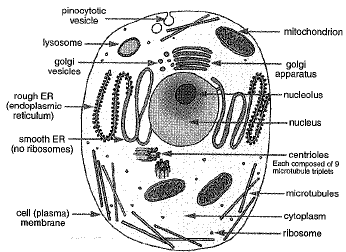Offer running on EduRev: Apply code STAYHOME200 to get INR 200 off on our premium plan EduRev Infinity!

135 docs

,

,

,

,

,

,

,

,

,

,

,

,

,

,

,

,

,

,

,

,

,

;